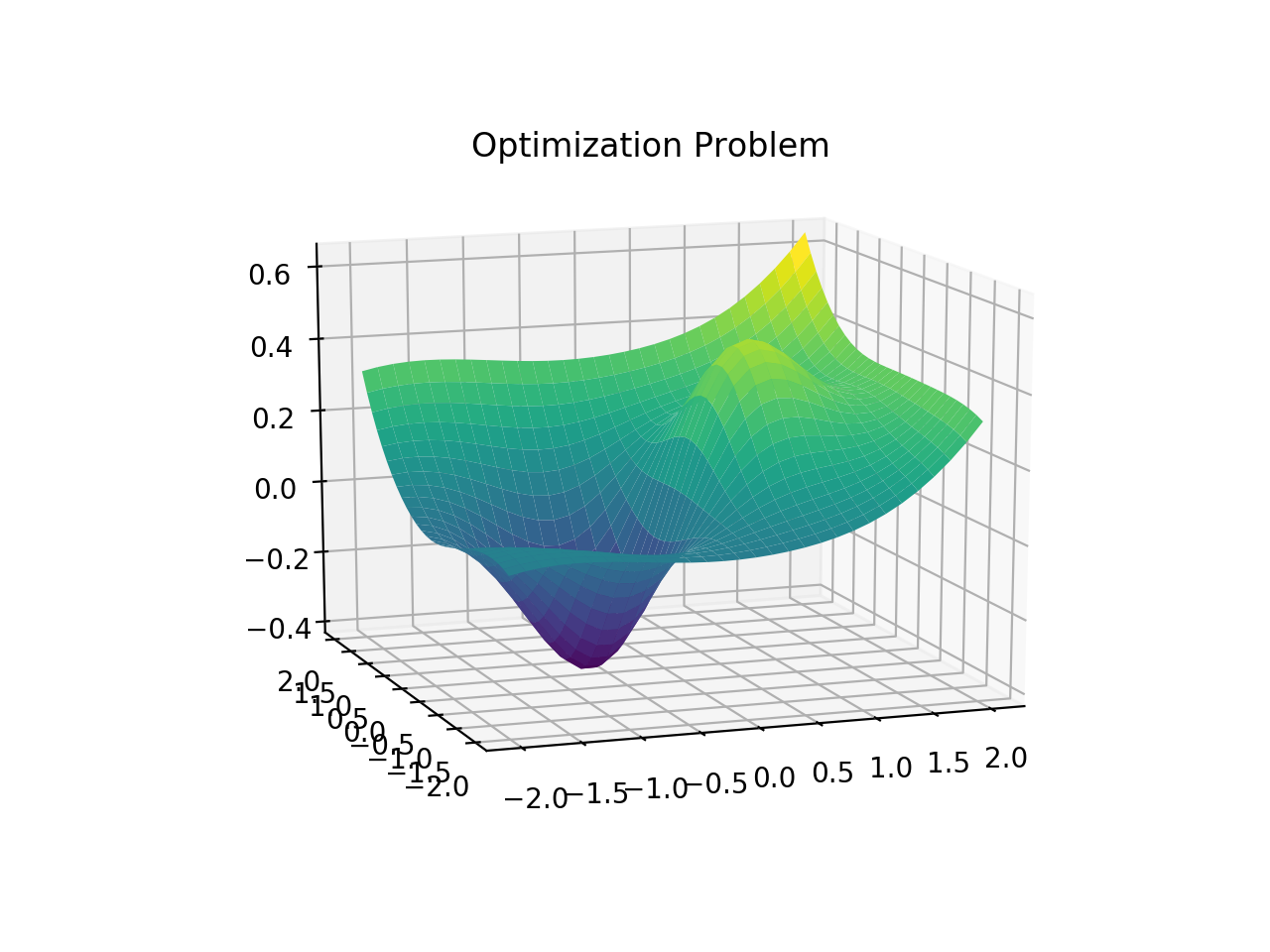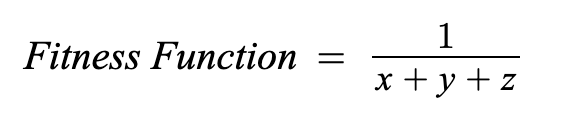1624496700

# Optimize Your Algorithms Tail Call Optimization

While writing code and algorithms you should consider tail call optimization (TCO).

### What is tail call optimization?

The tail call optimization is the fact of optimizing the recursive functions in order to avoid building up a tall call stack. You should as well know that some programming languages are doing tail call optimizations.

For example, Python and Java decided to don’t use TCO. While JavaScript allows to use TCO since ES2015-ES6.

Even if you know that your favorite language support natively TCO or not, I would definitely recommend you to assume that your compiler/interpreter will not do the work for you.

### How to do a tail call optimization?

There are two famous methods to do a tail call optimization and avoid tall call stacks.

### 1. Going bottom-up

As you know recursions are building up the call stack so if we avoid such recursions in our algorithms it will will allow us to save on the memory usage. This strategy is called the bottom-up (we start from the beginning, while a recursive algorithm starts from the end after building a stack and works backwards.)

Let’s take an example with the following code (top-down — recursive code):

``````function product1ToN(n) {
return (n > 1) ? (n * product1ToN(n-1)) : 1;
}
``````

As you can see this code has a problem: it builds up a call stack of size O(n), which makes our total memory cost O(n). This code makes us vulnerable to a stack overflow error, where the call stack gets too big and runs out of space.

In order to optimize our example we need to go bottom-down and remove the recursion:

``````function product1ToN(n) {
let result = 1;
for (let num = 1; num <= n; num++) {
result *= num;
}
return result;
}
``````

This time we are not stacking up our calls in the call stack, and we do use a O(1) space complexity(with a O(n) time complexity).

#memoization #programming #algorithms #optimization #optimize

## Buddha Community1624496700

## Optimize Your Algorithms Tail Call Optimization

While writing code and algorithms you should consider tail call optimization (TCO).

### What is tail call optimization?

The tail call optimization is the fact of optimizing the recursive functions in order to avoid building up a tall call stack. You should as well know that some programming languages are doing tail call optimizations.

For example, Python and Java decided to don’t use TCO. While JavaScript allows to use TCO since ES2015-ES6.

Even if you know that your favorite language support natively TCO or not, I would definitely recommend you to assume that your compiler/interpreter will not do the work for you.

### How to do a tail call optimization?

There are two famous methods to do a tail call optimization and avoid tall call stacks.

### 1. Going bottom-up

As you know recursions are building up the call stack so if we avoid such recursions in our algorithms it will will allow us to save on the memory usage. This strategy is called the bottom-up (we start from the beginning, while a recursive algorithm starts from the end after building a stack and works backwards.)

Let’s take an example with the following code (top-down — recursive code):

``````function product1ToN(n) {
return (n > 1) ? (n * product1ToN(n-1)) : 1;
}
``````

As you can see this code has a problem: it builds up a call stack of size O(n), which makes our total memory cost O(n). This code makes us vulnerable to a stack overflow error, where the call stack gets too big and runs out of space.

In order to optimize our example we need to go bottom-down and remove the recursion:

``````function product1ToN(n) {
let result = 1;
for (let num = 1; num <= n; num++) {
result *= num;
}
return result;
}
``````

This time we are not stacking up our calls in the call stack, and we do use a O(1) space complexity(with a O(n) time complexity).

#memoization #programming #algorithms #optimization #optimize1624867080

## Algorithm trading backtest and optimization examplesAlgorithm trading backtest and optimization examples.

### xbtusd-vanila-market-making-backtest-hedge1598775060

## Optimization Techniques: Genetic Algorithm

In complex machine learning models, the performance usually depends on multiple input parameters. In order to get the optimal model, the parameters must be properly tuned. However, when there are multiple parameter variables, each ranging across a wide spectrum of values, there are too many possible configurations for each set of parameters to be tested. In these cases, optimization methods should be used to attain the optimal input parameters without spending vast amounts of time finding them.In the diagram above, it shows the distribution of the model based on only two parameters. As evident in the example shown, it is not always an easy task to find the maximum or minimum of the curve. This is why optimization methods and algorithms are crucial in the field of machine learning.

## Genetic Algorithm

The most commonly used optimization strategy are Genetic Algorithms. Genetic Algorithms are based off of Darwin’s theory of natural selection. It is relatively easy to implement and there is a lot of flexibility for the setup of the algorithm so that it can be applied to a wide range of problems.

### Choosing a Fitness Function

To start off, there must be a fitness function that measures how well a set of input parameters perform. Solutions with a higher fitness derived from a fitness function will be better than ones with a lower fitness.

For example, if a solution has a cost of x + y + z, then the fitness function should try to minimize the cost. This can be done with the following fitness function#genetic-algorithm #optimization #genetics #optimization-algorithms #machine-learning1593362160

## Validate the Correctness of an Evolutionary Optimization Algorithm

1. Introduction
2. Correctness Validation
3. A Brief Discussion on Performance Evaluation
4. Conclusion
5. References
6. Some Related Medium Posts

# 1. Introduction

Evolutionary and Metaheuristic optimization algorithms are considered as a subset of Artificial Intelligence (AI). These algorithms try to imitate nature mechanisms and solve an optimization problem. For example, the Genetic Algorithm (GA) uses inspired biological mechanisms such as reproduction, cross-over, selection, mutation, and recombination to find the best solution for an optimization problem. Another example can be an Imperialist Competitive Algorithm (ICA) where social mechanisms like domination, revolution, and colonization are used to find a solution . Evolutionary algorithms are usually performing well in complex optimization problems where using classical approaches is mathematically difficult. They can easily deal with nonlinear problems without being stuck in local minimums.

To optimize a problem with an evolutionary optimization algorithm one can use a certified toolbox. However, for some algorithms, either there is no available certified toolbox or one may prefer to implement his/her own code with any program languages. In this situation, the most important questions are — How we can trust the written code? How we can validate the correctness of our implemented evolutionary or metaheuristic code?

In this post, some of the existing ways to check and validate the correctness of an implemented evolutionary optimization algorithm have been addressed briefly. In addition, a discussion regarding the performance evaluation of optimization algorithms is provided using an example of Ackley function.

# 2. Correctness Validation

There are different ways to check the correctness and accuracy of an implemented metaheuristic multi-objective optimization algorithm (e.g. Genetic Algorithm (GA) or Differential Evolution (DE)). The following approaches can be addressed briefly to check the correctness of the implemented optimization algorithm:

A) Comparison with classical approaches: In some problems, it can be possible to run both the classical approach and multi-variate GA to make sure the results are correct and accurate. However, in most cases, because of mathematical complexity using a classical approach for comparison is not possible.

B) Using Well-known Benchmarks: The correctness of the code can be also proven though existing benchmarks. The used algorithm can be validated via well-known benchmarks [2–6].

C) Using Expert Idea: An expert of the system can check the results and their rationality. They should all agree that the final results are logical. If for a problem exact minimum cost value is needed, then using an expert idea would not be convincing.

D) Convergence: The convergence of the implemented multi-objective genetic algorithm can be easily observed through the plot of cost vs. iterations. There are also other factors such as roundoff errors and local extrema that have the same perspective.

E) Domination Factor: The new generation of optimization algorithms such as NSGA-II, NSGA-III, and MOPSO usually have a function to separate dominant results from non-dominant one. So, all final results should be dominant and with a proper initial population, it is very rare to be trapped by a local minimum. However, there is no guarantee that they find the global minimum of the system. For more information regarding the domination please check .

#metaheuristics #artificial-intelligence #evolutionary-algorithms #optimization-algorithms #performance #algorithms1593350760

## Genetic Algorithm (GA): A Simple and Intuitive Guide

Learn what are metaheuristics and why we use them sometimes instead of traditional optimization algorithms. Learn the metaheuristic Genetic Algorithm (GA) and how it works through a simple step by step guide.

#genetic-algorithm #algorithms #optimization #metaheuristics #data-science #algorithms